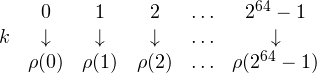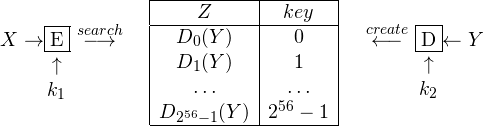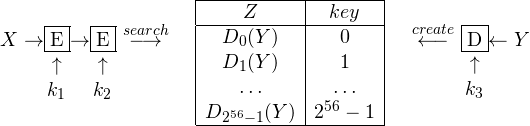# Meet-in-the-middle

One technique to strengthen ciphers is iteration. We have seen that all modern ciphers are based on rounds, i.e., repetitions of the same core algorithm. We might wonder what happens if we iterate a whole cipher such as DES or AES. There is at least a good reason for that: increasing key length. DES, for example, has a 56-bit key that is considered weak nowadays. If we iterate the cipher using a different key we obtain a key pair (k1,k2) of 112 bits which is, in principle, too hard to break (but we will see that this is not the case).

#### DES is non-idempotent

We know that iteration makes sense only if the cipher is not idempotent. The following informal argument suggests that modern ciphers are very unlikely to be idempotent. We reason on DES but the same reasoning would apply to different block ciphers.
DES has a block size of 64 bits. If we list all the 264 possible blocks and we pick one DES key, the cipher will map each of these blocks into a different block. Since encryption must be invertible, this mapping is injective. Thus, in any block cipher, a key corresponds to a permutation of all the possible plaintext blocks:So, for example, 0 (64 zero bits) is mapped to 3214112, 1 to 213210312421 and so on. Now, the number of permutations of 264 elements is 264! which is enormously big compared to the 256 DES keys. We reason as follows: the way a DES key selects a specific permutation is “complex” (otherwise the cipher would be weak). We can thus think of DES keys as selecting a random subset of 256 permutations among the 264! possible ones. Now, the probability that the composition of two such permutations is still in this subset is, intuitively, 256 / 264! which is a very small, negligible number. This means that it is really unlikely that 2 iterations of DES (and of any modern block cipher, in fact) correspond to a single encryption under a different key.

As far as DES is concerned, it has been formally proved that it is not idempotent [CW92].

#### Meet-in-the-middle

We thus consider DoubleDES (2DES), i.e., the iteration of DES twice.
Is it really true that now, in order to break the cipher, we have to try all the possible key pairs?
The answer is ‘NO’. We can do better by exploiting the so called Meet-in-the-middle attack. It is a known-plaintext scenario, i.e., the attacker knows pair of plaintext/ciphertext (X,Y), (X’,Y’), (X”,Y”), … all encrypted under the same key K. The idea is to select one pair, say (X,Y), and try to decrypt Y with all the possible second keys k2. All the resulting values Z are stored into a table together with the key, which is indexed by Z. Now we try to encrypt under all the possible first keys k1 the plaintext X and we look the obtained value into the table. If we find a match we test the resulting pair (k1,k2) on all the other plaintext/ciphertext pairs and, if all the tests succeeds, we give it as output. The attack is illustrated below:Pseudo-code follows:

table=emptyTable()
for all k2:    # step1: build the table
table.append(dec(k2,Y), k2)

for all k1:    # step2: try all first keys
k2 = search(table,enc(k1,X))
if k2:     # if ciphertext is in the table
for all pairs (X',Y'):
check enc(k2,enc(k1,X'))=Y'
if all checks succeeded:
return(k1,k2)


The computational cost of this attack is 257 steps and 256 space. In fact, first step takes 256 steps to build a table which is 256 entries. Second step takes at most 256 steps to find the right key. We thus have 256 + 256 = 257 steps.

False keys. It is very important that, whenever a pair (k1,k2) for (X,Y) is found, it is tested against other pairs (X’,Y’). It could be the case, in fact, that a key pair is fine for (X,Y) but it is not the right key pair. This can happen more frequently than expected. To estimate the number of these false keys we assume that plaintexts are mapped to ciphertext uniformly by the possible keys. In other words, the number of keys mapping X into Y is approximatively the same as the number of keys mapping X into any other ciphertext Y’. This assumption typically holds for any good cipher for which observing Y gives very little information about the plaintext X. Having a non-uniform distribution would imply that the plaintexts mapped by more keys into Y are more likely than the ones mapped by less keys.

Under this assumption, we can then estimate the number of false keys as |K||C|, i.e., the number of keys divided by the number of ciphertexts which is, for 2DES, 2112/264= 248. This huge number of possible keys encrypting X into Y can be reduced very quickly by testing keys on more pairs. The probability that a false key is also OK for (X’,Y’) is just 1 over the number of all the possible ciphertexts (we have only one good case Y’ over all the possible 264 ciphertexts) giving 1264. Thus, the number of false keys is reduced to 248264 = 1216. If we try on one more pair we get 1280, and so on. In summary, with 3 available pairs of plaintext/ciphertext we can run the attack having a negligible probability of getting a false key.

#### Lesson learned

The cost in time is thus basically the same as the one for a single iteration of DES. For this reason, 2DES is never used in practice and, instead, we have a triple iteration known as triple-DES (3DES). This gives a 168-bit triple key (k1,k2,k3). The meet-in-the-middle attack is still possible but it reduces the cost in time to 2112 with a table of size 256 entries. The idea is to build the table by decrypting Y under all k3 and then try all the pairs (k1,k2), as illustrated below: